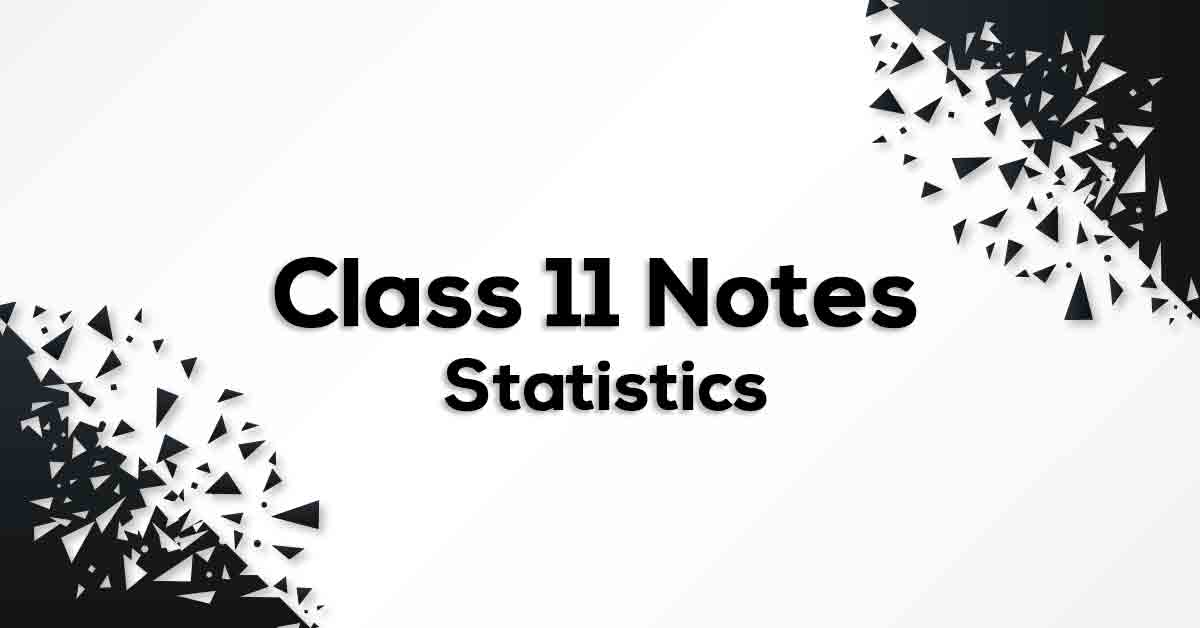Statistics Class 11 Formulas & Notes | Vidyakul
×

# Statistics Class 11 Formulas & Notes## Class 11 Math Chapter 15 Statistics Formulas & Notes - PDF Download

Statistics Class 11 Formulas & Notes are cumulated in a systematic manner which gets rid of confusion among children regarding the course content since CBSE keeps on updating the course every year. The formulae list covers all formulae which provides the students a simple way to study of revise the chapter. Statistics Class 11 Formulas & Notes would provide you with an effective preparation for your examinations.

### How do you define Statistics?

Statistics forms the basis of analyzing numbers. The data set can be raw, grouped and ungrouped. In this chapter, we’ll be studying collecting raw data, representing data in the form of histogram, bar graph or the frequency of polygon and how to find mean, median and mode.